# Estimation of weighted data¶

Elan Ding
Modified: June 10, 2018

Github Repository

In this post, I will show you how to obtain statistically accurate point and interval estimates from weighted survey data. Most survey documentations have it that directly analyzing a complex survey without taking into consideration the sample weights will lead to inaccurate results.

Here we will look at the National Health and Nutrition Examination Survey (NHANES) dataset, and try to obtain point and interval estimates of cholesterol levels based on race, ethnicity, and socioeconomic status.

## 1: Collecting NHANES data from 2003-2016¶

### 1.1 Basic Information¶

The datasets used for the analysis are provided in the Github repository from above. The original data is in .XPT format, which is used for SAS. Using a Python module xport, I converted them into .csv format using

python -m xport example.xpt > example.csv

### 1.2 Data Collection¶

We read the data using Pandas first. If you need more information about each files, you can check out the Github Repository or NHANES.

In :
import numpy as np
import pandas as pd
import matplotlib.pyplot as plt
import seaborn as sns
%matplotlib inline

In :
# Demographics

# HDL

hdl03 = pd.read_csv('L13_C.csv') # also contains total cholesterol in 2003

# Triglyceride

trig15 = pd.read_csv('BIOPRO_I.csv') # triglyceride in 2015

# Total Cholesterol (2003 data is included in hdl03)



Since the NHANES 2015 dataset does not have the adjusted weights for Triglyceride levels, we use MEC weights from the DEMO_I_15 file, adjusted by a factor 4/5. (Reason will be shown below.) See survey guidelines.

In :
trig15['WTSAF2YR'] = demo15['WTMEC2YR']*4/5


Next, we combine data files from 2003-2016 based on categories.

In :
# Demographics

demo = pd.concat([demo03, demo05, demo07, demo09, demo11, demo13, demo15], sort=False)
demo = demo[['SEQN','RIAGENDR', 'RIDAGEYR', 'RIDRETH1','DMDEDUC3', 'DMDEDUC2',
'INDFMINC','INDFMIN2','WTINT2YR','WTMEC2YR']]
demo.columns = ['Index', 'Gender', 'Age', 'Ethnicity', 'Education 6-19', 'Education 20+',
'Income 2003-05', 'Income 2007+', 'Weight Int', 'Weight MEC']
demo.set_index('Index', inplace = True)

# Combine columns

demo['Education 6-19'] = demo['Education 6-19'].fillna(0.0)
demo['Education 20+'] = demo['Education 20+'].fillna(0.0)
demo['Income 2003-05'] = demo['Income 2003-05'].fillna(0.0)
demo['Income 2007+'] = demo['Income 2007+'].fillna(0.0)
demo['Education'] = demo['Education 6-19'] + demo['Education 20+']
demo['Income'] = demo['Income 2003-05'] + demo['Income 2007+']
demo.drop(['Education 6-19', 'Education 20+', 'Income 2003-05', 'Income 2007+'], axis=1, inplace = True)

In :
# HDL

hdl = pd.concat([hdl03, hdl05, hdl07, hdl09, hdl11, hdl13, hdl15], sort=False)
hdl = hdl[['SEQN', 'LBDHDD', 'LBXHDD']]
hdl.columns = ['Index', 'HDL1', 'HDL2']
hdl.set_index('Index', inplace = True)

# Combine columns

hdl['HDL1'] = hdl['HDL1'].fillna(0.0)
hdl['HDL2'] = hdl['HDL2'].fillna(0.0)
hdl['HDL'] = hdl['HDL1'] + hdl['HDL2']
hdl.drop(['HDL1', 'HDL2'], axis=1, inplace=True)

In :
# Tryglyceride

trig = pd.concat([trig03, trig05, trig07, trig09, trig11, trig13, trig15], sort=False)
trig = trig[['SEQN', 'WTSAF2YR', 'LBXTR', 'LBXSTR']]
trig.columns = ['Index', 'Weight Fast', 'Triglyceride', 'Triglyceride2']
trig.set_index('Index', inplace = True)

# Combine columns

trig['Triglyceride'] = trig['Triglyceride'].fillna(0.0)
trig['Triglyceride2'] = trig['Triglyceride2'].fillna(0.0)
trig['Triglyceride'] = trig['Triglyceride'] + trig['Triglyceride2']
trig.drop(['Triglyceride2'], axis=1, inplace=True)

In :
# Total Cholesterol

tchol = pd.concat([hdl03, tchol05, tchol07, tchol09, tchol11, tchol13, tchol15], sort=False)
tchol = tchol[['SEQN', 'LBXTC']]
tchol.columns = ['Index', 'Total Chol']
tchol.set_index('Index', inplace = True)

In :
# Combine all into one data frame

from functools import reduce

dfs = [demo, hdl, trig, tchol]
df = reduce(lambda left,right: left.join(right), dfs)
df = df[['Age', 'Gender', 'Ethnicity', 'Education', 'Income', 'HDL',
'Triglyceride', 'Total Chol', 'Weight Int', 'Weight MEC', 'Weight Fast']]

Out:
Index 21005.0 21006.0 21007.0 21008.0 21009.0
Age 19.000000 16.000000 14.000000 17.000000 55.000000
Gender 1.000000 2.000000 2.000000 1.000000 1.000000
Ethnicity 4.000000 4.000000 3.000000 4.000000 3.000000
Education 11.000000 11.000000 8.000000 10.000000 3.000000
Income 6.000000 6.000000 6.000000 7.000000 8.000000
HDL 44.000000 53.000000 69.000000 66.000000 37.000000
Triglyceride 102.000000 71.000000 NaN NaN NaN
Total Chol 189.000000 159.000000 212.000000 175.000000 254.000000
Weight Int 5512.320949 5422.140453 39764.177412 5599.499351 97593.678977
Weight MEC 5824.782465 5564.039715 40591.066325 5696.750596 97731.727244
Weight Fast 14084.200000 13081.000000 NaN NaN NaN
In :
df['Age'].describe()

Out:
count    71058.000000
mean        31.198204
std         24.850422
min          0.000000
25%          9.000000
50%         25.000000
75%         51.750000
max         85.000000
Name: Age, dtype: float64

Using seaborn's heatmap, we can check for missing values.

In :
# Check for missing values (Yellow bar indicades missing value)

sns.heatmap(df.isnull(), yticklabels = False, cbar=False, cmap = 'viridis')

Out:
<matplotlib.axes._subplots.AxesSubplot at 0x10f001908>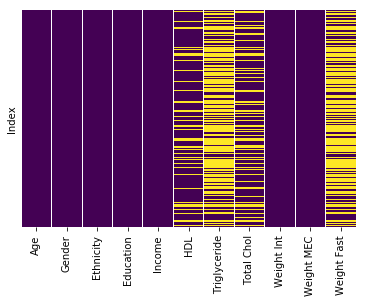## 2 Designing sample weights¶

According to NHANES guidelines, there are three types of weights, with different uses.

• WTINT2YR - (Weight Int) This should be used for analyzing interview data in a 2-year cycle , such as demographic information.

• WTMEC2YR - (Weight MEC) This weight should be used for analyzing MEC examinination data in a 2-year cycle, such as HDL levels.

• WTSAF2YR - (Weight Fast) This subsample weight should be used for analyzing Triglyceride levels.

First we look at the raw weights, and note that they sum to the size of U.S. population. For example, in 2005, the raw weights sum to roughly 290 million, which is roughly the U.S. population in 2005.

In :
demo05['WTINT2YR'].sum()

Out:
291616891.83997804

Note that the fasting subsample weight sums to less than US population. (This is more than 4/5 of the U.S. population. This is the reason we adjusted the MEC weight by 4/5 earlier.)

In :
trig05['WTSAF2YR'].sum()

Out:
243600773.577

### 2.1 Combining survey cycles¶

to change the survey weigths after combining the 7 2-year cycles (2003-2004, 2005-2006, 2007-2008, 2009-2010, 2011-2012 2013-2014, 2015-2016). Let $\{w_1,w_2,...,w_n\}$ be the original weights of the combined dataset. The adjusted weights are defined by $$w'_i = \frac{1}{7} w_i, \,\, i=1,...,n$$

In :
df['Weight Int'] = df['Weight Int']/7
df['Weight MEC'] = df['Weight MEC']/7
df['Weight Fast'] = df['Weight Fast']/7

Out:
Index 21005.0 21006.0 21007.0 21008.0 21009.0
Age 19.000000 16.000000 14.000000 17.000000 55.000000
Gender 1.000000 2.000000 2.000000 1.000000 1.000000
Ethnicity 4.000000 4.000000 3.000000 4.000000 3.000000
Education 11.000000 11.000000 8.000000 10.000000 3.000000
Income 6.000000 6.000000 6.000000 7.000000 8.000000
HDL 44.000000 53.000000 69.000000 66.000000 37.000000
Triglyceride 102.000000 71.000000 NaN NaN NaN
Total Chol 189.000000 159.000000 212.000000 175.000000 254.000000
Weight Int 787.474421 774.591493 5680.596773 799.928479 13941.954140
Weight MEC 832.111781 794.862816 5798.723761 813.821514 13961.675321
Weight Fast 2012.028571 1868.714286 NaN NaN NaN

### 2.2 Normalizing weights¶

Let $\{S_1,S_2,...,S_n\}$ be the original sample of size $n$, associated with raw weights $\{w_1,w_2,...,w_n\}$.

For each sampled unit $S_i$, define the normalized sample weight as $$w'_i = \frac{w_i}{\frac{1}{n} \sum_{i=1}^n w_i}.$$

In this case the sum of the normalized weights sum to the sample size: $$\sum_{i=1}^n w'_i = \frac{\sum_{i=1}^n w_i}{\frac{1}{n} \sum_{j=1}^n w_j} = n$$

In :
df['Weight Int'] = df['Weight Int']/df['Weight Int'].mean()
df['Weight MEC'] = df['Weight MEC']/df['Weight MEC'].mean()
df['Weight Fast'] = df['Weight Fast']/df['Weight Fast'].mean()

Out:
Index 21005.0 21006.0 21007.0 21008.0 21009.0
Age 19.000000 16.000000 14.000000 17.000000 55.000000
Gender 1.000000 2.000000 2.000000 1.000000 1.000000
Ethnicity 4.000000 4.000000 3.000000 4.000000 3.000000
Education 11.000000 11.000000 8.000000 10.000000 3.000000
Income 6.000000 6.000000 6.000000 7.000000 8.000000
HDL 44.000000 53.000000 69.000000 66.000000 37.000000
Triglyceride 102.000000 71.000000 NaN NaN NaN
Total Chol 189.000000 159.000000 212.000000 175.000000 254.000000
Weight Int 0.185532 0.182497 1.338371 0.188466 3.284780
Weight MEC 0.196049 0.187273 1.366202 0.191740 3.289426
Weight Fast 0.227178 0.210996 NaN NaN NaN
In :
df['Weight MEC'].sum()

Out:
71058.0

Let $\{S_1,S_2,...,S_n\}$ be a sample of size $n$, with normalized weights $\{w_1, w_2,...,w_n\}$, such that $\sum_{i=1}^n w_i = n$.

Let $\{U_1,U_2,...,U_k\}, k\leq n$ be a subsample with weights $\{w'_1, w'_2,...,w'_n\}$, which are defined as $$w'_i = \frac{w_i}{\frac{1}{k}\sum_{j=1}^k w_j}$$ Note that the sum of the adjusted sample weights sum to $k$: $$\sum_{i=1}^k w'_i = \frac{\sum_{i=1}^k w_i}{\frac{1}{k}\sum_{j=1}^k w_j} = k.$$ This method will be applied each time we take a sub-sample of the 71058 individuals in our combined dataset. Next, we take subsamples based on HDL, Trigylceride, and Total Cholesterol.

#### 2.3.1 HDL Subsample¶

In :
# Taking a subsample of college students age 18-24
df_hdl = df[(df['Age'] > 17) & (df['Age'] < 25) &
(df['Education'].isin([3.0, 4.0, 5.0, 13.0, 14.0, 15.0]))
][['Age', 'Gender', 'Ethnicity', 'Income', 'HDL', 'Weight MEC']].copy()

# Check for missing values
sns.heatmap(df_hdl.isnull(), yticklabels = False, cbar=False, cmap = 'viridis')

Out:
<matplotlib.axes._subplots.AxesSubplot at 0x10d1a1eb8>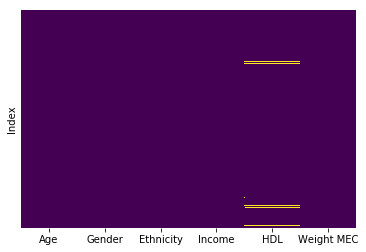In :
# Fill in the missing value by taking a sample average:
df_hdl['HDL'].fillna(df_hdl['HDL'].mean(), inplace=True)

df_hdl['Weight MEC'] = df_hdl['Weight MEC']/df_hdl['Weight MEC'].mean()

# Note that the updated weights sum to the sub-sample size:
df_hdl['Weight MEC'].sum()

Out:
4370.0

#### 2.3.2 Triglyceride Subsample¶

In :
# Taking a subsample of college students age 18-24
df_trig = df[(df['Age'] > 17) & (df['Age'] < 25) &
(df['Education'].isin([3.0, 4.0, 5.0, 13.0, 14.0, 15.0]))
][['Age', 'Gender', 'Ethnicity', 'Income', 'Triglyceride', 'Weight Fast']].copy()

# Check for missing values
sns.heatmap(df_trig.isnull(), yticklabels = False, cbar=False, cmap = 'viridis')

Out:
<matplotlib.axes._subplots.AxesSubplot at 0x1a10573e80>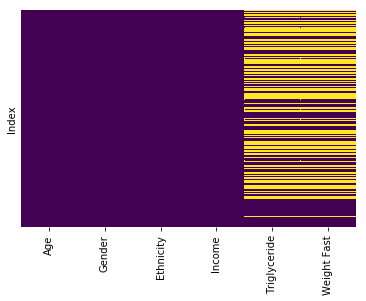In :
# Note that the missing values occur together, so we drop them:
df_trig = df_trig.dropna()

df_trig['Weight Fast'] = df_trig['Weight Fast']/df_trig['Weight Fast'].mean()

# Note that the updated weights sum to the sub-sample size:
df_trig['Weight Fast'].sum()

Out:
2338.0

#### 2.3.3 Total Cholesterol Subsample¶

In :
# Taking a subsample of college students age 18-24
df_tchol = df[(df['Age'] > 17) & (df['Age'] < 25) &
(df['Education'].isin([3.0, 4.0, 5.0, 13.0, 14.0, 15.0]))
][['Age', 'Gender', 'Ethnicity', 'Income', 'Total Chol', 'Weight MEC']].copy()

# Check for missing values
sns.heatmap(df_tchol.isnull(), yticklabels = False, cbar=False, cmap = 'viridis')

Out:
<matplotlib.axes._subplots.AxesSubplot at 0x1a166deb38>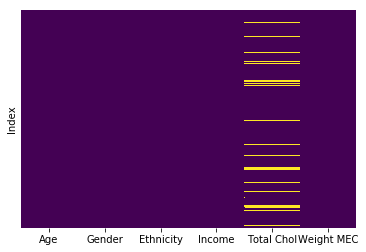In :
# Fill in the missing value by taking a sample average:
df_tchol['Total Chol'] = df_tchol['Total Chol'].fillna(df_tchol['Total Chol'].mean())

df_tchol['Weight MEC'] = df_tchol['Weight MEC']/df_tchol['Weight MEC'].mean()

# Note that the updated weights sum to the sub-sample size:
df_tchol['Weight MEC'].sum()

Out:
4370.0

## 3 Data Analysis¶

Now we have three clean data sets df_hdl, df_trig, df_tchol. We are finally to do some data analysis!

### 3.1 Remapping data¶

First we divide "Income" into three groups:

1. "low" - if Family Income < 20000
2. "mid" - if 20000 < Family Income < 75000
3. "high" - if Family Income > 75000


We also specify the ethnicity values according to NHANES guide:

1. Mexican
2. Hispanic
3. White
4. Black
5. Other


Fianlly, we apply the map for gender:

1. Male
2. Female
In :
# Mapping income and ethnicity

def income_map( x ):
if x in [1.0, 2.0, 3.0, 4.0, 13.0]:
return 'low'
elif x in [5.0, 6.0, 7.0, 8.0, 9.0, 10.0, 12.0]:
return 'mid'
elif x in [11.0, 14.0, 15.0]:
return 'high'
else:
return np.nan

ethnicity_map = {1:'Mexican', 2:'Hispanic', 3:'White', 4:'Black', 5:'Other'}
gender_map = {1.0:'Male', 2.0: 'Female'}

df_hdl['Income'] = df_hdl['Income'].apply(income_map)
df_trig['Income'] = df_trig['Income'].apply(income_map)
df_tchol['Income'] = df_tchol['Income'].apply(income_map)

df_hdl['Gender'] = df_hdl['Gender'].map(gender_map)
df_trig['Gender'] = df_trig['Gender'].map(gender_map)
df_tchol['Gender'] = df_tchol['Gender'].map(gender_map)

df_hdl['Ethnicity'] = df_hdl['Ethnicity'].map(ethnicity_map)
df_trig['Ethnicity'] = df_trig['Ethnicity'].map(ethnicity_map)
df_tchol['Ethnicity'] = df_tchol['Ethnicity'].map(ethnicity_map)


### 3.2 Mathematical Background¶

For iid sample points $X_1, X_2,...,X_n$, it is fairly straight forward to find the sample mean and sample variance by \begin{aligned} \bar{X} &= \frac{1}{n}\sum X_i \\ S^2 &= \frac{1}{n-1}\sum (X_i-\bar{X})^2 \end{aligned}

However, if each $X_i$ is associated with a weight $w_i$, the old estimate does not apply anymore. (Consider the extreme case where $X_1$ has weight 1 and $X_2,...,X_n$ have weights 0.)

#### 3.2.1 Calculating Weighted Sample Variance¶

Let $X_1, X_2,..., X_n$ be samples with sample weights $w_1,w_2,...,w_n$. Then define $$p_i = \frac{w_i}{\sum_{i=1}^n w_i}, \quad \text{ such that } \sum_{i=1}^n p_i = 1$$

The weighted sample mean is naturally defined by $$\bar{X} = \sum_{i=1}^n X_i p_i$$ The biased weighted sample variance is given by $$S_{\text{bias}}^2 = \sum_{i=1}^n (X_i - \bar{X})^2 p_i = \sum_{i=1}^n X_i^2 p_i - \bar{X}^2$$

This variance estimate is biased. For small sample size, we need to make adjustments.

#### 3.2.2 Adjusting for weighted sample variance biase¶

To adjust for the biase in $S_{\text{bias}}^2$, we take the expected value: \begin{aligned} E(S_{\text{bias}}^2) &= E\left[\sum_{i=1}^n(X_i - \mu)^2 p_i - 2(\bar{X}-\mu)\sum_{i=1}^n(X_i - \mu)p_i + (\bar{X}-\mu)^2\sum_{i=1}^n p_i \right] \\ &=E\left[\sum_{i=1}^n(X_i-\mu)^2p_i - 2(\bar{X}-\mu)\left(\sum_{i=1}^n(X_i-\bar{X})p_i + \sum_{i=1}^n(\bar{X}-\mu)p_i\right) + (\bar{X}-\mu)^2 \right] \\ &=E\left[ \sum_{i=1}^n(X_i-\mu)^2 p_i - 2(\bar{X}-\mu)^2 + (\bar{X}-\mu)^2\right] \\ &=E\left[\sum_{i=1}^n (X_i-\mu)^2 p_i - (\bar{X}-\mu)^2\right] \\ &=E\left[\sum_{i=1}^n (X_i-\mu)^2 p_i\right] - E\left[(\bar{X}-\mu)^2\right] \\ &=\sum_{i=1}^np_i E\left[(X_i-\mu)^2\right] - \text{Var}(\bar{X}) + \left[E(\bar{X}-\mu)\right]^2 \\ &= \sigma^2 - \text{Var}\left[\sum_{i=1}^n X_i p_i\right] \\ &= \sigma^2\left(1-\sum_{i=1}^np_i^2\right) \end{aligned}

Hence, we define the unbiased weighted sample variance as $$S^2 = \frac{1}{1-\sum_{i=1}^n p_i^2} \sum_{i=1}^n(X_i-\bar{X})^2 p_i = \left(1- \frac{\sum_{i=1}^n w_i^2}{\left(\sum_{i=1}^n w_i\right)^2}\right)^{-1}\left(\sum_{i=1}^n X_i^2 p_i - \bar{X}^2\right)$$

If we are using normalized weights, then $\sum_{i=1}^n w_i = n$, so that $$S^2 = \left(1- \frac{1}{n^2} \sum_{i=1}^n w_i^2\right)^{-1}\left(\sum_{i=1}^n X_i^2 p_i - \bar{X}^2\right)$$

We write a Python function that outputs three numbers $(a,b,c)$ where $a$ is the point estimate of the mean, and $(b,c)$ is the $100(1-\alpha)\%$ confidence interval. For this analysis, we use $\alpha=0.05$ to be the significance level.

In :
# Define a function that retuns (a, b, c) where a is the point estimate of the mean,
# and (b, c) is the 100*(1-alpha)% confidence interval

import scipy.stats as st

def weighted_estimate( values, weights, alpha ):

mean = np.average( values, weights = weights )
S = np.sqrt((np.average( values**2, weights = weights ) - mean**2 )
/( 1-np.sum(weights**2)/len(weights)**2) )

return (mean, mean - st.t.ppf(1-alpha/2, len(weights)-1)*S/np.sqrt(len(weights)),
mean + st.t.ppf(1-alpha/2, len(weights)-1)*S/np.sqrt(len(weights)) )


We are now ready to apply our method to each data sets separately.

### 3.3 HDL Analysis¶

In :
df_hdl.head()

Out:
Age Gender Ethnicity Income HDL Weight MEC
Index
21097.0 23.0 Female Black mid 45.0 0.775859
21107.0 20.0 Male White mid 29.0 1.860274
21130.0 20.0 Female Black high 53.0 0.806247
21171.0 20.0 Female Black low 58.0 0.894561
21175.0 18.0 Female Black low 53.0 0.184017
In :
df_hdl['HDL'].count()

Out:
4370

#### 3.3.1 Gender¶

In :
f, ax = plt.subplots(figsize=(10, 2))
sns.boxplot(y='Gender', x='HDL', data=df_hdl, palette='coolwarm')
sns.despine(trim=True, left=True)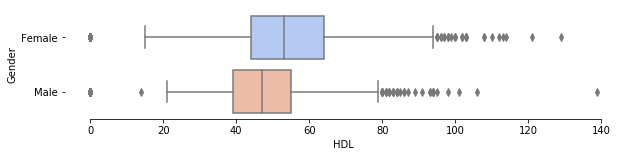In :
df_hdl.groupby('Gender')['HDL'].describe()

Out:
count mean std min 25% 50% 75% max
Gender
Female 2304.0 51.768137 20.818749 0.0 44.0 53.0 64.0 129.0
Male 2066.0 44.927618 18.023397 0.0 39.0 47.0 55.0 139.0
In :
hdl_values_male = np.array(df_hdl[df_hdl['Gender'] == 'Male']['HDL'])
hdl_weights_male = np.array(df_hdl[df_hdl['Gender'] == 'Male']['Weight MEC'] /
df_hdl[df_hdl['Gender'] == 'Male']['Weight MEC'].mean())

hdl_values_female = np.array(df_hdl[df_hdl['Gender'] == 'Female']['HDL'])
hdl_weights_female = np.array(df_hdl[df_hdl['Gender'] == 'Female']['Weight MEC'] /
df_hdl[df_hdl['Gender'] == 'Female']['Weight MEC'].mean())

In :
weighted_estimate(hdl_values_female, hdl_weights_female, 0.05)

Out:
(51.61787802850586, 50.751461153280545, 52.48429490373117)
In :
weighted_estimate(hdl_values_male, hdl_weights_male, 0.05)

Out:
(44.104081667381, 43.32438701352597, 44.883776321236034)

#### 3.3.2 Income¶

In :
f, ax = plt.subplots(figsize=(10, 2))
sns.boxplot(y='Income', x='HDL', data=df_hdl, palette='coolwarm')
sns.despine(trim=True, left=True)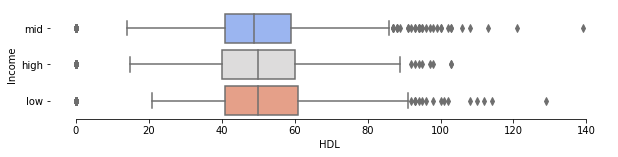In :
df_hdl.groupby('Income')['HDL'].describe()

Out:
count mean std min 25% 50% 75% max
Income
high 745.0 48.325749 20.177194 0.0 40.0 50.0 60.0 103.0
low 1521.0 49.046533 20.035971 0.0 41.0 50.0 61.0 129.0
mid 1902.0 48.346778 19.469684 0.0 41.0 49.0 59.0 139.0
In :
hdl_values_low = np.array(df_hdl[df_hdl['Income'] == 'low']['HDL'])
hdl_weights_low = np.array(df_hdl[df_hdl['Income'] == 'low']['Weight MEC'] /
df_hdl[df_hdl['Income'] == 'low']['Weight MEC'].mean())

hdl_values_mid = np.array(df_hdl[df_hdl['Income'] == 'mid']['HDL'])
hdl_weights_mid = np.array(df_hdl[df_hdl['Income'] == 'mid']['Weight MEC'] /
df_hdl[df_hdl['Income'] == 'mid']['Weight MEC'].mean())

hdl_values_high = np.array(df_hdl[df_hdl['Income'] == 'high']['HDL'])
hdl_weights_high = np.array(df_hdl[df_hdl['Income'] == 'high']['Weight MEC'] /
df_hdl[df_hdl['Income'] == 'high']['Weight MEC'].mean())

In :
weighted_estimate(hdl_values_low, hdl_weights_low, 0.05)

Out:
(48.314575234559996, 47.284023264745315, 49.34512720437468)
In :
weighted_estimate(hdl_values_mid, hdl_weights_mid, 0.05)

Out:
(48.08472537260012, 47.20433232307273, 48.96511842212751)
In :
weighted_estimate(hdl_values_high, hdl_weights_high, 0.05)

Out:
(47.03794813680216, 45.592474225654044, 48.48342204795027)

#### 3.3.3 Ethnicity¶

In :
f, ax = plt.subplots(figsize=(10, 3))
sns.boxplot(y='Ethnicity', x='HDL', data=df_hdl, palette='coolwarm')
sns.despine(trim=True, left=True)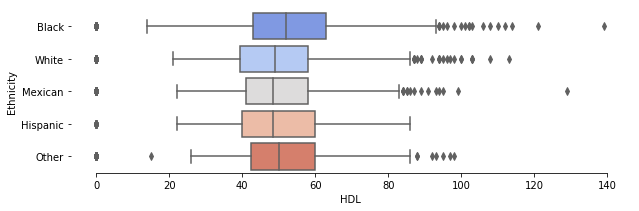In :
df_hdl.groupby('Ethnicity')['HDL'].describe()

Out:
count mean std min 25% 50% 75% max
Ethnicity
Black 1137.0 50.406463 21.553712 0.0 43.00 52.000000 63.0 139.0
Hispanic 333.0 48.291533 17.720432 0.0 40.00 48.534153 60.0 86.0
Mexican 771.0 48.346070 18.258124 0.0 41.00 48.534153 58.0 129.0
Other 519.0 48.950454 19.104403 0.0 42.50 50.000000 60.0 98.0
White 1610.0 47.217958 19.870374 0.0 39.25 49.000000 58.0 113.0
In :
hdl_values_black = np.array(df_hdl[df_hdl['Ethnicity'] == 'Black']['HDL'])
hdl_weights_black = np.array(df_hdl[df_hdl['Ethnicity'] == 'Black']['Weight MEC'] /
df_hdl[df_hdl['Ethnicity'] == 'Black']['Weight MEC'].mean())

hdl_values_white = np.array(df_hdl[df_hdl['Ethnicity'] == 'White']['HDL'])
hdl_weights_white = np.array(df_hdl[df_hdl['Ethnicity'] == 'White']['Weight MEC'] /
df_hdl[df_hdl['Ethnicity'] == 'White']['Weight MEC'].mean())

hdl_values_mexican = np.array(df_hdl[df_hdl['Ethnicity'] == 'Mexican']['HDL'])
hdl_weights_mexican = np.array(df_hdl[df_hdl['Ethnicity'] == 'Mexican']['Weight MEC'] /
df_hdl[df_hdl['Ethnicity'] == 'Mexican']['Weight MEC'].mean())

hdl_values_hispanic = np.array(df_hdl[df_hdl['Ethnicity'] == 'Hispanic']['HDL'])
hdl_weights_hispanic = np.array(df_hdl[df_hdl['Ethnicity'] == 'Hispanic']['Weight MEC'] /
df_hdl[df_hdl['Ethnicity'] == 'Hispanic']['Weight MEC'].mean())

hdl_values_other = np.array(df_hdl[df_hdl['Ethnicity'] == 'Other']['HDL'])
hdl_weights_other = np.array(df_hdl[df_hdl['Ethnicity'] == 'Other']['Weight MEC'] /
df_hdl[df_hdl['Ethnicity'] == 'Other']['Weight MEC'].mean())

In :
weighted_estimate(hdl_values_black, hdl_weights_black, 0.05)

Out:
(50.56182728500764, 49.283717911154284, 51.83993665886099)
In :
weighted_estimate(hdl_values_white, hdl_weights_white, 0.05)

Out:
(46.97077309995158, 45.986118494488984, 47.95542770541417)
In :
weighted_estimate(hdl_values_mexican, hdl_weights_mexican, 0.05)

Out:
(48.096380786432746, 46.83398746210728, 49.35877411075821)
In :
weighted_estimate(hdl_values_hispanic, hdl_weights_hispanic, 0.05)

Out:
(48.11521101439338, 46.21901053918815, 50.011411489598615)
In :
weighted_estimate(hdl_values_other, hdl_weights_other, 0.05)

Out:
(49.9491658137644, 48.25059701634863, 51.64773461118018)

### Triglyceride Analysis¶

In :
df_trig.head()

Out:
Age Gender Ethnicity Income Triglyceride Weight Fast
Index
21107.0 20.0 Male White mid 143.0 2.482447
21130.0 20.0 Female Black high 64.0 1.207012
21219.0 21.0 Male White mid 92.0 2.482447
21221.0 23.0 Male Mexican mid 101.0 1.160214
21313.0 22.0 Female White low 100.0 0.000000
In :
df_trig['Triglyceride'].count()

Out:
2338

#### 3.3.1 Gender¶

In :
f, axes = plt.subplots(2, 1, figsize=(10, 4))
sns.boxplot(y='Gender', x='Triglyceride', data=df_trig, palette='coolwarm', ax=axes)
sns.boxplot(y='Gender', x='Triglyceride', data=df_trig, palette='coolwarm', ax=axes, showfliers=False)
sns.despine(trim=True, left=True)
axes.set_title("Hiding Outliers")
f.tight_layout()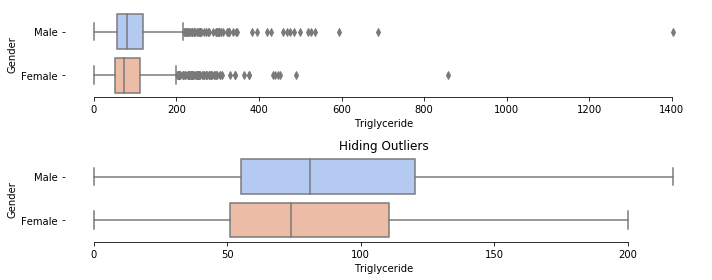In :
df_trig.groupby('Gender')['Triglyceride'].describe()

Out:
count mean std min 25% 50% 75% max
Gender
Female 1242.0 87.856683 67.503332 0.0 51.0 74.0 110.75 857.0
Male 1096.0 98.790146 85.898399 0.0 55.0 81.0 120.25 1403.0
In :
trig_values_male = np.array(df_trig[df_trig['Gender'] == 'Male']['Triglyceride'])
trig_weights_male = np.array(df_trig[df_trig['Gender'] == 'Male']['Weight Fast'] /
df_trig[df_trig['Gender'] == 'Male']['Weight Fast'].mean())

trig_values_female = np.array(df_trig[df_trig['Gender'] == 'Female']['Triglyceride'])
trig_weights_female = np.array(df_trig[df_trig['Gender'] == 'Female']['Weight Fast'] /
df_trig[df_trig['Gender'] == 'Female']['Weight Fast'].mean())

In :
weighted_estimate(trig_values_female, trig_weights_female, 0.05)

Out:
(93.77461046558993, 90.19344874126992, 97.35577218990994)
In :
weighted_estimate(trig_values_male, trig_weights_male, 0.05)

Out:
(106.67718450402494, 102.41275573168058, 110.9416132763693)

#### 3.3.2 Income¶

In :
f, axes = plt.subplots(2, 1, figsize=(10, 5))
sns.boxplot(y='Income', x='Triglyceride', data=df_trig, palette='coolwarm', ax=axes)
sns.boxplot(y='Income', x='Triglyceride', data=df_trig, palette='coolwarm', ax=axes, showfliers=False)
sns.despine(trim=True, left=True)
axes.set_title("Hiding Outliers")
f.tight_layout()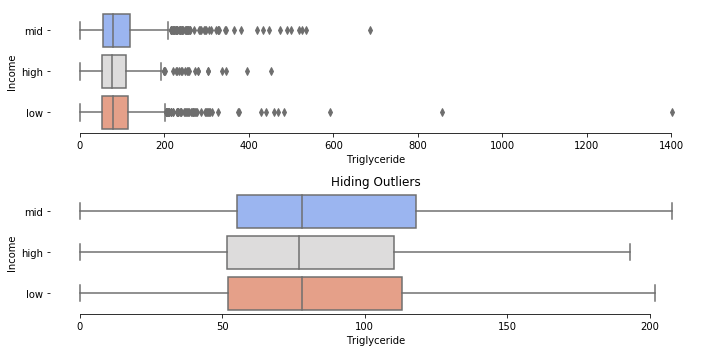In :
df_trig.groupby('Income')['Triglyceride'].describe()

Out:
count mean std min 25% 50% 75% max
Income
high 408.0 87.693627 63.854655 0.0 51.75 77.0 110.25 452.0
low 792.0 94.069444 88.720311 0.0 52.00 78.0 113.00 1403.0
mid 1021.0 95.343781 72.917482 0.0 55.00 78.0 118.00 688.0
In :
trig_values_low = np.array(df_trig[df_trig['Income'] == 'low']['Triglyceride'])
trig_weights_low = np.array(df_trig[df_trig['Income'] == 'low']['Weight Fast'] /
df_trig[df_trig['Income'] == 'low']['Weight Fast'].mean())

trig_values_mid = np.array(df_trig[df_trig['Income'] == 'mid']['Triglyceride'])
trig_weights_mid = np.array(df_trig[df_trig['Income'] == 'mid']['Weight Fast'] /
df_trig[df_trig['Income'] == 'mid']['Weight Fast'].mean())

trig_values_high = np.array(df_trig[df_trig['Income'] == 'high']['Triglyceride'])
trig_weights_high = np.array(df_trig[df_trig['Income'] == 'high']['Weight Fast'] /
df_trig[df_trig['Income'] == 'high']['Weight Fast'].mean())

In :
weighted_estimate(trig_values_low, trig_weights_low, 0.05)

Out:
(105.39334440455805, 99.62061794069714, 111.16607086841896)
In :
weighted_estimate(trig_values_mid, trig_weights_mid, 0.05)

Out:
(97.86760914226276, 93.92223216332383, 101.8129861212017)
In :
weighted_estimate(trig_values_high, trig_weights_high, 0.05)

Out:
(99.52404621515471, 94.126219089902, 104.92187334040743)

#### 3.3.3 Ethnicity¶

In :
f, axes = plt.subplots(2, 1, figsize=(10, 6))
sns.boxplot(y='Ethnicity', x='Triglyceride', data=df_trig, palette='coolwarm', ax=axes)
sns.boxplot(y='Ethnicity', x='Triglyceride', data=df_trig, palette='coolwarm', ax=axes, showfliers=False)
sns.despine(trim=True, left=True)
axes.set_title("Hiding Outlier")
f.tight_layout()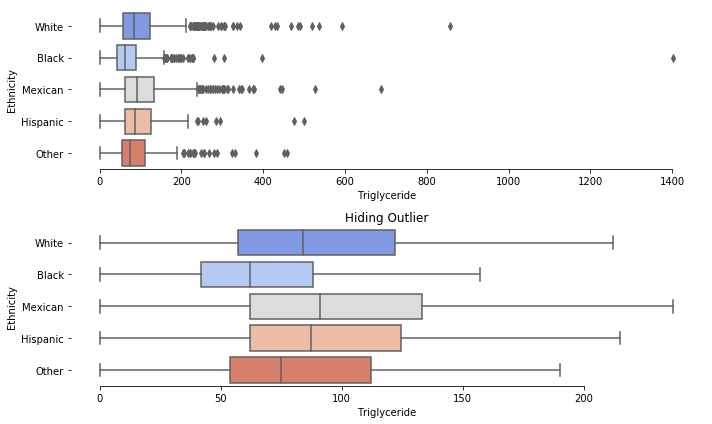In :
df_trig.groupby('Ethnicity')['Triglyceride'].describe()

Out:
count mean std min 25% 50% 75% max
Ethnicity
Black 600.0 70.823333 72.170468 0.0 42.00 62.0 88.0 1403.0
Hispanic 198.0 100.005051 66.897945 0.0 62.00 87.5 124.5 500.0
Mexican 424.0 110.514151 82.051819 0.0 62.00 91.0 133.0 688.0
Other 304.0 91.154605 68.028574 0.0 53.75 75.0 112.0 459.0
White 812.0 99.172414 79.187099 0.0 57.00 84.0 122.0 857.0
In :
trig_values_black = np.array(df_trig[df_trig['Ethnicity'] == 'Black']['Triglyceride'])
trig_weights_black = np.array(df_trig[df_trig['Ethnicity'] == 'Black']['Weight Fast'] /
df_trig[df_trig['Ethnicity'] == 'Black']['Weight Fast'].mean())

trig_values_white = np.array(df_trig[df_trig['Ethnicity'] == 'White']['Triglyceride'])
trig_weights_white = np.array(df_trig[df_trig['Ethnicity'] == 'White']['Weight Fast'] /
df_trig[df_trig['Ethnicity'] == 'White']['Weight Fast'].mean())

trig_values_mexican = np.array(df_trig[df_trig['Ethnicity'] == 'Mexican']['Triglyceride'])
trig_weights_mexican = np.array(df_trig[df_trig['Ethnicity'] == 'Mexican']['Weight Fast'] /
df_trig[df_trig['Ethnicity'] == 'Mexican']['Weight Fast'].mean())

trig_values_hispanic = np.array(df_trig[df_trig['Ethnicity'] == 'Hispanic']['Triglyceride'])
trig_weights_hispanic = np.array(df_trig[df_trig['Ethnicity'] == 'Hispanic']['Weight Fast'] /
df_trig[df_trig['Ethnicity'] == 'Hispanic']['Weight Fast'].mean())

trig_values_other = np.array(df_trig[df_trig['Ethnicity'] == 'Other']['Triglyceride'])
trig_weights_other = np.array(df_trig[df_trig['Ethnicity'] == 'Other']['Weight Fast'] /
df_trig[df_trig['Ethnicity'] == 'Other']['Weight Fast'].mean())

In :
weighted_estimate(trig_values_black, trig_weights_black, 0.05)

Out:
(75.07059328308932, 71.55001903253421, 78.59116753364444)
In :
weighted_estimate(trig_values_white, trig_weights_white, 0.05)

Out:
(105.86121933411441, 100.74662523292787, 110.97581343530095)
In :
weighted_estimate(trig_values_mexican, trig_weights_mexican, 0.05)

Out:
(111.29989261594667, 104.67611322845559, 117.92367200343776)
In :
weighted_estimate(trig_values_hispanic, trig_weights_hispanic, 0.05)

Out:
(104.45806207209937, 95.52479330708967, 113.39133083710907)
In :
weighted_estimate(trig_values_other, trig_weights_other, 0.05)

Out:
(88.57341958647942, 82.58431425635916, 94.56252491659967)

### Total Cholesterol Analysis¶

In :
df_tchol.head()

Out:
Age Gender Ethnicity Income Total Chol Weight MEC
Index
21097.0 23.0 Female Black mid 165.0 0.775859
21107.0 20.0 Male White mid 152.0 1.860274
21130.0 20.0 Female Black high 191.0 0.806247
21171.0 20.0 Female Black low 171.0 0.894561
21175.0 18.0 Female Black low 146.0 0.184017
In :
df_tchol['Total Chol'].count()

Out:
4370

#### 3.3.1 Gender¶

In :
f, ax = plt.subplots(figsize=(10, 2))
sns.boxplot(y='Gender', x='Total Chol', data=df_tchol, palette='coolwarm')
sns.despine(trim=True, left=True)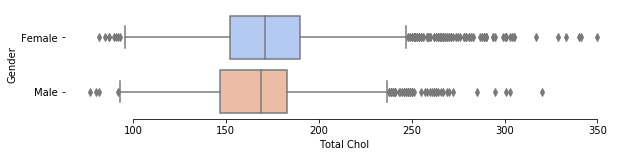In :
df_tchol.groupby('Gender')['Total Chol'].describe()

Out:
count mean std min 25% 50% 75% max
Gender
Female 2304.0 174.276422 34.814598 82.0 152.0 170.916515 190.0 350.0
Male 2066.0 167.169553 30.496788 77.0 147.0 169.000000 183.0 320.0
In :
tchol_values_male = np.array(df_tchol[df_tchol['Gender'] == 'Male']['Total Chol'])
tchol_weights_male = np.array(df_tchol[df_tchol['Gender'] == 'Male']['Weight MEC'] /
df_tchol[df_tchol['Gender'] == 'Male']['Weight MEC'].mean())

tchol_values_female = np.array(df_tchol[df_tchol['Gender'] == 'Female']['Total Chol'])
tchol_weights_female = np.array(df_tchol[df_tchol['Gender'] == 'Female']['Weight MEC'] /
df_tchol[df_tchol['Gender'] == 'Female']['Weight MEC'].mean())

In :
weighted_estimate(tchol_values_female, tchol_weights_female, 0.05)

Out:
(174.49210547907865, 173.07329414882048, 175.9109168093368)
In :
weighted_estimate(tchol_values_male, tchol_weights_male, 0.05)

Out:
(168.79403465076132, 167.44352335895744, 170.1445459425652)

#### 3.3.2 Income¶

In :
f, ax = plt.subplots(figsize=(10, 2))
sns.boxplot(y='Income', x='Total Chol', data=df_tchol, palette='coolwarm')
sns.despine(trim=True, left=True)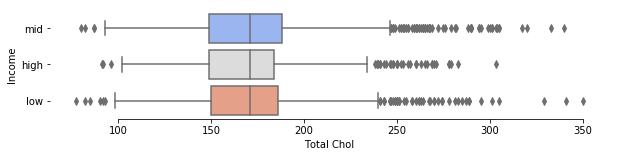In :
df_tchol.groupby('Income')['Total Chol'].describe()

Out:
count mean std min 25% 50% 75% max
Income
high 745.0 170.657141 31.916580 91.0 149.0 170.916515 184.0 303.0
low 1521.0 170.560688 32.710198 77.0 150.0 170.916515 186.0 350.0
mid 1902.0 171.551816 34.052889 80.0 149.0 170.916515 188.0 340.0
In :
tchol_values_low = np.array(df_tchol[df_tchol['Income'] == 'low']['Total Chol'])
tchol_weights_low = np.array(df_tchol[df_tchol['Income'] == 'low']['Weight MEC'] /
df_tchol[df_tchol['Income'] == 'low']['Weight MEC'].mean())

tchol_values_mid = np.array(df_tchol[df_tchol['Income'] == 'mid']['Total Chol'])
tchol_weights_mid = np.array(df_tchol[df_tchol['Income'] == 'mid']['Weight MEC'] /
df_tchol[df_tchol['Income'] == 'mid']['Weight MEC'].mean())

tchol_values_high = np.array(df_tchol[df_tchol['Income'] == 'high']['Total Chol'])
tchol_weights_high = np.array(df_tchol[df_tchol['Income'] == 'high']['Weight MEC'] /
df_tchol[df_tchol['Income'] == 'high']['Weight MEC'].mean())

In :
weighted_estimate(tchol_values_low, tchol_weights_low, 0.05)

Out:
(171.23113467737096, 169.57906516251504, 172.88320419222688)
In :
weighted_estimate(tchol_values_mid, tchol_weights_mid, 0.05)

Out:
(172.04877771718307, 170.52165305052532, 173.57590238384083)
In :
weighted_estimate(tchol_values_high, tchol_weights_high, 0.05)

Out:
(171.64714382381223, 169.3226212533826, 173.97166639424185)

#### 3.3.3 Ethnicity¶

In :
f, ax = plt.subplots(figsize=(10, 3))
sns.boxplot(y='Ethnicity', x='Total Chol', data=df_tchol, palette='coolwarm')
sns.despine(trim=True, left=True)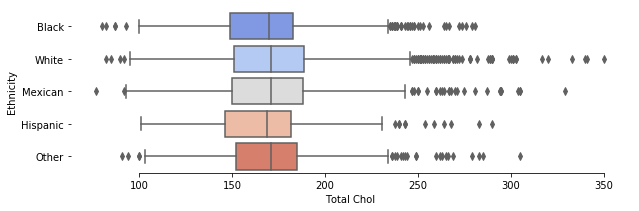In :
df_tchol.groupby('Ethnicity')['Total Chol'].describe()

Out:
count mean std min 25% 50% 75% max
Ethnicity
Black 1137.0 168.453076 30.636016 80.0 149.0 170.000000 183.0 281.0
Hispanic 333.0 167.029763 30.427093 101.0 146.0 169.000000 182.0 290.0
Mexican 771.0 171.519657 34.134899 77.0 150.0 170.916515 188.0 329.0
Other 519.0 171.243880 31.024265 91.0 152.0 170.916515 185.0 305.0
White 1610.0 173.065767 35.060863 82.0 151.0 170.916515 189.0 350.0
In :
tchol_values_black = np.array(df_tchol[df_tchol['Ethnicity'] == 'Black']['Total Chol'])
tchol_weights_black = np.array(df_tchol[df_tchol['Ethnicity'] == 'Black']['Weight MEC'] /
df_tchol[df_tchol['Ethnicity'] == 'Black']['Weight MEC'].mean())

tchol_values_white = np.array(df_tchol[df_tchol['Ethnicity'] == 'White']['Total Chol'])
tchol_weights_white = np.array(df_tchol[df_tchol['Ethnicity'] == 'White']['Weight MEC'] /
df_tchol[df_tchol['Ethnicity'] == 'White']['Weight MEC'].mean())

tchol_values_mexican = np.array(df_tchol[df_tchol['Ethnicity'] == 'Mexican']['Total Chol'])
tchol_weights_mexican = np.array(df_tchol[df_tchol['Ethnicity'] == 'Mexican']['Weight MEC'] /
df_tchol[df_tchol['Ethnicity'] == 'Mexican']['Weight MEC'].mean())

tchol_values_hispanic = np.array(df_tchol[df_tchol['Ethnicity'] == 'Hispanic']['Total Chol'])
tchol_weights_hispanic = np.array(df_tchol[df_tchol['Ethnicity'] == 'Hispanic']['Weight MEC'] /
df_tchol[df_tchol['Ethnicity'] == 'Hispanic']['Weight MEC'].mean())

tchol_values_other = np.array(df_tchol[df_tchol['Ethnicity'] == 'Other']['Total Chol'])
tchol_weights_other = np.array(df_tchol[df_tchol['Ethnicity'] == 'Other']['Weight MEC'] /
df_tchol[df_tchol['Ethnicity'] == 'Other']['Weight MEC'].mean())

In :
weighted_estimate(tchol_values_black, tchol_weights_black, 0.05)

Out:
(168.79054389349733, 166.99352315362904, 170.58756463336562)
In :
weighted_estimate(tchol_values_white, tchol_weights_white, 0.05)

Out:
(172.72829722569105, 171.07199210088245, 174.38460235049965)
In :
weighted_estimate(tchol_values_mexican, tchol_weights_mexican, 0.05)

Out:
(170.35639821328928, 168.08613341910083, 172.62666300747773)
In :
weighted_estimate(tchol_values_hispanic, tchol_weights_hispanic, 0.05)

Out:
(167.85462846789065, 164.3232148624996, 171.3860420732817)
In :
weighted_estimate(tchol_values_other, tchol_weights_other, 0.05)

Out:
(172.7579045095308, 169.9165864098209, 175.59922260924068)

## 3 Results¶

The results can be summarized into the following table. Overall, we find that there is no significant differene in total cholesterol and HDL choleteral in terms of race and socioecnomic status. However, African-Americans have significantly lower triglyceride levels. Gender is a significant factor in all types of cholesterol levels.